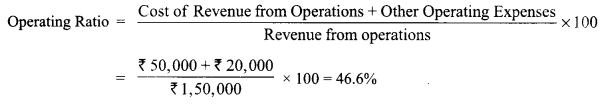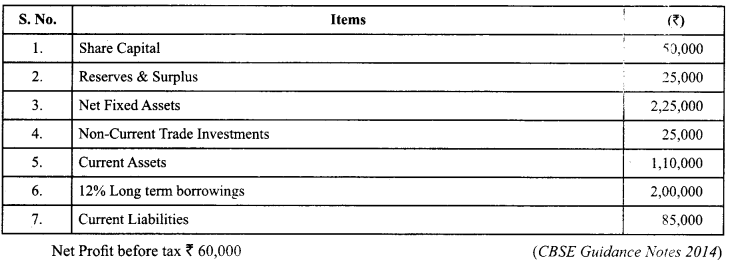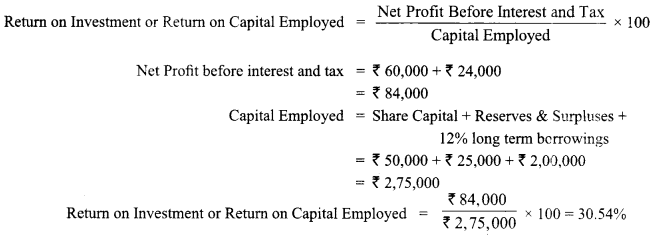Here we are providing Class 12 Accountancy Important Extra Questions and Answers Chapter 10 Accounting Ratios. Accountancy Class 12 Important Questions and Answers are the best resource for students which helps in class 12 board exams.

## Class 12 Accountancy Chapter 10 Important Extra Questions Accounting Ratios

### Accounting Ratios Important Extra Questions Very Short Answer Type

Question 1.
What will be the effect on current ratio if a bills payable is discharged on maturity? (CBSE SP 2019-20)
The current ratio will increase

Question 2.
Debt Equity Ratio of a company is 1:2. Purchase of a Fixed asset for ₹ 5,00,000 on long term deferred payment basis will increase, decrease or not change the ratio?
Increased

Question 3.
It is a simple arithmetical expression of relationship between two figures. Name the term.
Ratio

Question 4.
The liquidity of a business firm is measured by its ability to satisfy its long-term obligations as they become due. Name a ratio used for this purpose.
Current Ratio.

Question 5.
X Ltd. has a Debt-Equity Ratio at 3 : 1. According to the management it should be maintained at 1 : 1. What is the choice to do so?
To increase the equity or reduce the debt.

Question 6.
How the solvency of a business is assessed by Financial Statement Analysis? (CBSE Delhi 2012)
With the help of solvency ratios.

Question 7.
Assuming that the debt to equity ratio is 1 : 2, state giving reason, whether the ratio will improve, decline or will have no change in case equity shares are issued for cash. (CBSE Foreign 2006)
Decrease.

Question 8.
Debt to equity ratio of a company is 08 : 1. State whether long term loan obtained by the company will increase, decrease or not change the ratio. (CBSE Outside Delhi 2008)
Increase.

Question 9.
Inventory Turnover ratio of a company is 3 times. State, giving reason, whether the ratio improve, decline or do not change because of increase in the value of closing stock by ₹ 5,000. (CBSE Outside Delhi 2008)
Decrease.

Question 10.
Trade Receivables Turnover Ratio of a company is 6 times. State with reason whether the ratio will improve, decrease or not change due to increase in the value of closing inventory by ₹ 50,000. (CBSE Foreign 2008)
No change. .

Question 11.
If a company has earned ₹ 10,00,000 as profit before interest and tax, ROI is 20%. State the capital employed in the company.
₹ 5,00,000Question 12.
What will be operating profit if operating ratio is 88.94? (CBSE Delhi 2009)
Operating Profit = 100 – 88.94 = 11.06

Question 13.
State with reason whether repayment of long-term loan will result in increase, decrease or no change of debt- equity ratio. (CBSE Outside Delhi 2010 Compt.)
Decrease.

Question 14.
A company has Share Capital of ₹ 5,00,000, Reserves and Surplus of ₹ 2,00,000 and Debt Equity Ratio of 1.8 : 1. It has issued additional Share Capital of ₹ 2,00,000 for cash and bonus shares of₹ 1,00,000. What will be new Debt Equity Ratio?
1.4 : 1

### Accounting Ratios Important Extra Questions Short Answer Type

Question 1.
(a) Calculate Revenue from operations of BN Ltd. From the following information: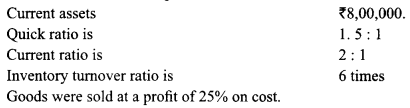Goods were sold at a profit of 25% on cost.
(b) The Operating ratio of a company is 60%. State whether ‘Purchase of goods costing ₹20,000’ will increase, decrease or not change the operating ratio. (CBSE Delhi 2019)
(a) Current Assets ₹8,00,000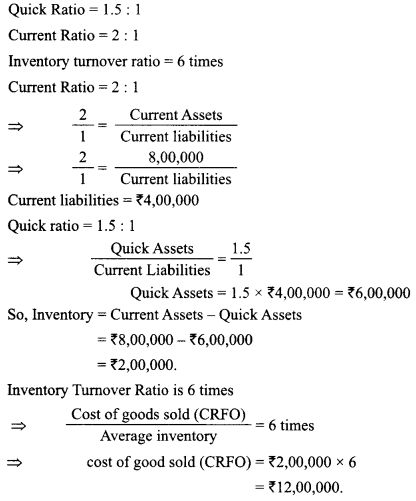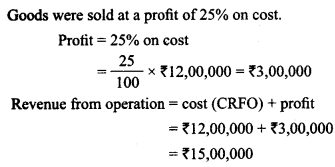(b) The operating ratio will not change, as there will be equal increase in purchases and closing inventory and hence cost of revenue from operation will remain unchanged.

Question 2.
(a) Calculate ‘Total Assets to Debt ratio’ from the following information: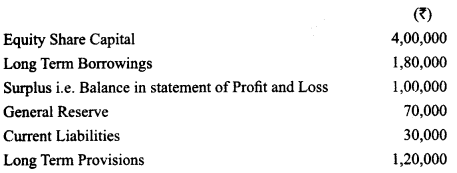(b) The Debt Equity ratio of a company is 1:2. State whether ‘Issue of bonus shares’ will increase, decrease or not change the Debt Equity Ratio.
a)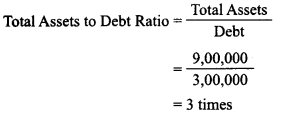Where Total Assets = Total liabilities
= Share capital + long-term borrowings + surplus + General Reserve + Current Liabilities +Long-term provisions
₹ 4,00,000 + ₹ 1 ,80,000 + ₹ 1,00,000+ ₹ 70,000 + ₹ 30,000 + ₹ 1,20,000
= ₹ 9,00,000
Debt = Long-term borrowings + long-term provision
= ₹ 1,80,000 + ₹ 1,20,000
₹ 3,00,000
b) Debt equity ratio of a company will not change due to issue of bonus shares, as neither the debt not equity is effected because R & S is converting into share capital.

Question 3.
The operating ratio of a company is 80%. State whether the following transactions will increase, decrease or not change the ratio :
(i) Purchased goes in íedit ₹ 20,000
(ii) Paid wae ₹ 5000
(iii) Redeemed ₹ 8000. 9% debentures
(iv) Sold goods ₹ 50,000 for cash

 S.No. Transactions Effect 1. Purchase goods on credit ₹ 20,000 No change 2. Paid wages ₹ 5,000 No change 3. Redeemed ₹ 8,000,9% Debentures No change 4. Sold goods ₹ 50,000 for cash No change

Question 4.
From the following information of Shiva Ltd., calculate total assets to debt ratio : (CBSE Outside Delhi 2019)Total Assets to Debts Ratio = $$\frac { Total Assets }{ Long Term Debt }$$
= 15,40,000/3,00,000 = 5.13
Total Assets = Fixed Assets + Non Current investments + Currents Assets
= ₹ 15,40,000
Debt = Total Liabilities – Equity Share Capital – Preference Share Capital – Reserves & Surplus – Current Liabilities = 3,00.000

Question 5.
From the given information, calculate the following ratios
(i) Operating Ratio
(ii) Inventory Turnover Ratio Information: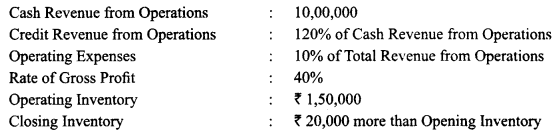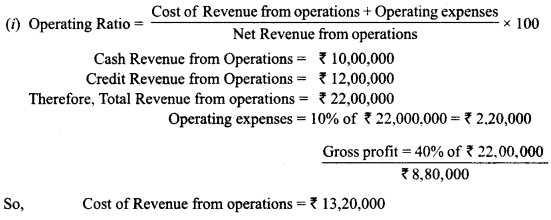Question 6.
(a) Net profit after interest and tax of M Ltd. was ₹ 1,00,000. Its Current Assets were ₹ 4,00,000 and Current Liabilities were ₹ 2,00,000. Tax rate was 50%. Its Total Assets were ₹ 10,00,000 and 10% Long term debt was ₹ 4,00,000.
Calculate Return of Investment.

(b) Rate of Gross profit on Revenue from operations of a company is 25%. Its Gross profit is ₹ 5,00,000. Its Shareholders’ Funds are ₹ 25,00,000; Non-current Liabilities are ₹ 8,00,000 and Non-current Assets are ₹ 23,00,000.
Calculate its Working Capital Turnover Ratio.
(a) Return on Investment = $$\frac { Profit before interest and tax }{ Capital employed }$$ x 100
Profit before interest and tax = ₹1,00,000 + ₹1,00,000 + ₹40,000
= ₹240,000
Capital employed = ₹8,00,000
Therefore, Return on Investment = ₹2,40,000/₹ 80,00,000 x 100
= 30%

(b) Working Capital Turnover ratio = Revenue from operations/Working Capital
Gross Profit = ₹5,00,000
So, Revenue from operations = ₹20,00,000
Working Capital = Shareholders Funds + Non Current liabilities – Non Current Assets
= ₹25,00,000 + ₹80,00,000 – ₹2300,000
= 10,00,000
Working Capital Turnover ratio = ₹20,00,000/₹ 10,00,000 = 2 times

Question 7.
(a) From the following details, calculate opening inventory: Closing inventory ₹ 60,000; Total Revenue from operations ₹ 5,00,000 (including cash revenue from operations ₹ 1,00,000); Total purchases ₹ 3,00,000 (including credit purchases ₹ 60,000). Goods are sold at a profit of 25% on cost.
(b) Current Assets of a company are 17,00.000. Its current ratio is 2.5 and Liquid ratio is 0.95. Calculate Current Liabilities and Inventory.
(a)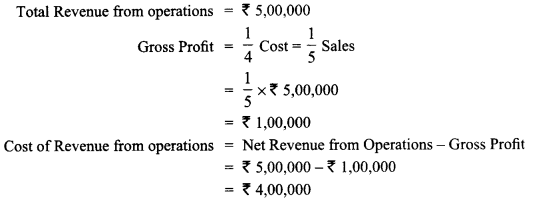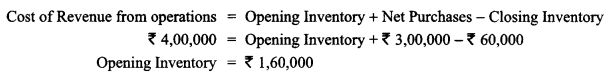(b)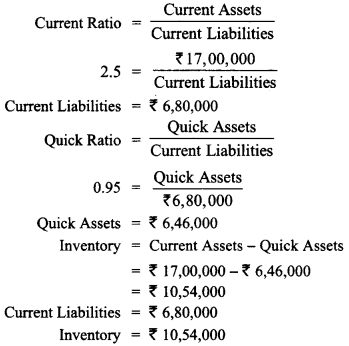Question 8.
Find the value of current liabilities and current assets, if current Ratio is 2.5 : 1, liquid ratio is 1.2 : 1 and the value of inventory of the firm is ₹ 78,000.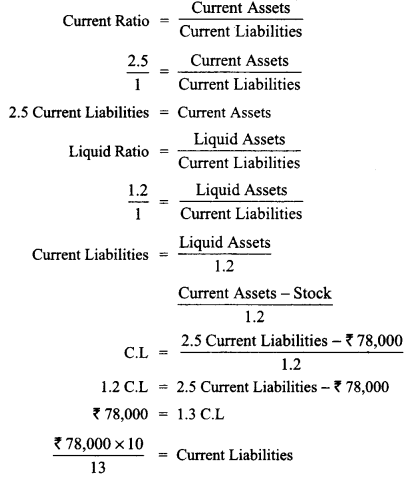₹ 60,000 = Current Liabilities
Current Assets = 2.5 x ₹ 60,000
=₹ 1,50,000

Question 9.
From the following compute (a) Current Ratio (b) Quick Ratio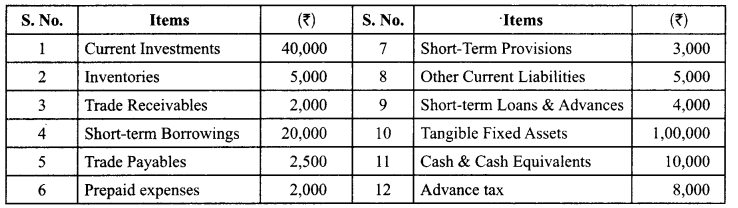(a)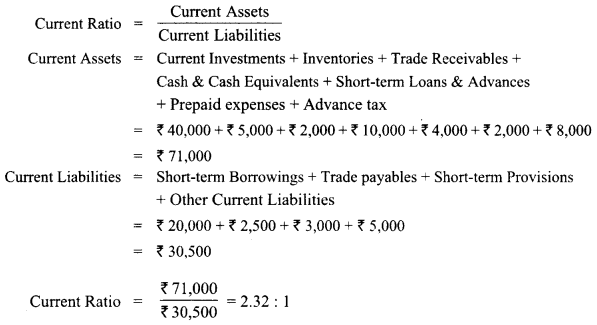(b)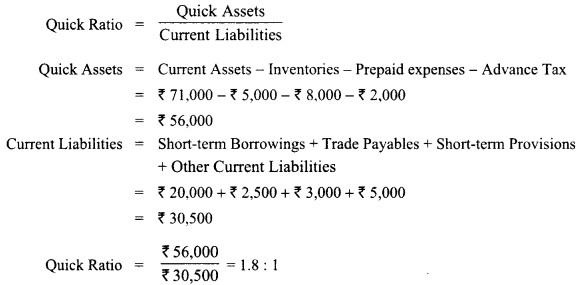Question 10.
From the following compute Current Ratio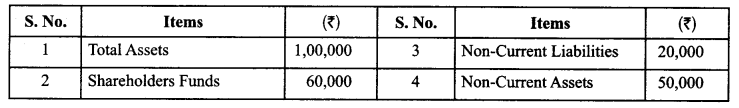Question 11.
(i) What is meant by solvency of business?
(ii) From the following details obtained from the financial statements of Jeev Ltd., calculate interest coverage ratio:
Net Profit after tax ₹ 1,20,000,
12% Long-term Debt ₹ 20,00,000,
Tax Rate 40%
(i) Solvency is the ability of a company to meet its long term financial obligations and. the interest on due
dates.Question 12.
Akshara Ltd. has 8% Debentures of ₹ Interest Coverage Ratio.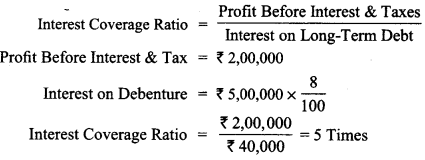Question 13.
From the following information related toNaveen Ltd. calculate
(a) Return on Investment and
(b) Total Assets to Debt Ratio.
Information: Fixed Assets 75,00,000; Current Assets ₹ 40,00,000; Current Liabilities ₹27,00,000; 12% Debentures ₹80,00,000 and Net Profit before Interest, Tax and Dividend ₹ 14,50,000. (Delhi 2015)Question 14.
From the following compute:
(a) Debt to Equity Ratio
(b) Total Assets to Debt Ratio
(c) Proprietary Ratio(a)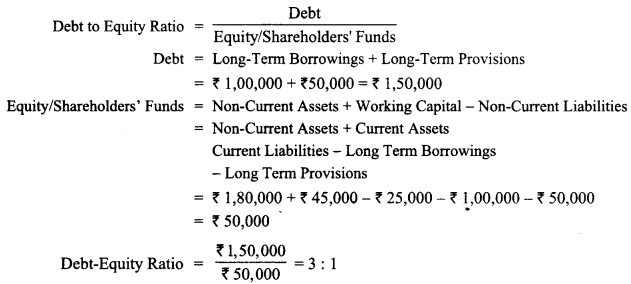Question 15.
Assuming that the Debt-Equity Ratio is 2:1, state giving reasons which of the following transactions would . (i) Increase; (ii) Decrease; Not alter the Debt-Equity Ratio:
(i) Issue of new shares for cash
(ii) Conversion of debentures into equity shares.
(iii) Sale of a fixed asset at profit.
(iv) Purchase of a fixed asset on long-term deferred payment basis.
(v) Payment to creditors (CBSE Guidance Notes 2014)
Statement showing the effect of various transactions on Debt-Equity Ratio.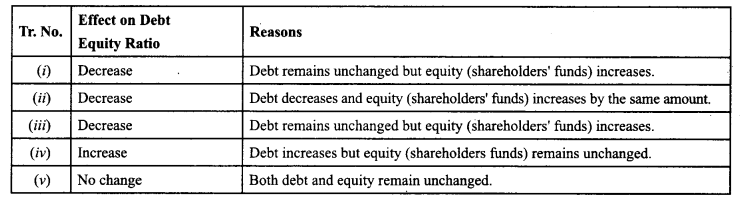Question 16.
Calculate amount of Opening Trade Receivables and Closing Trade Receivables from the following figures: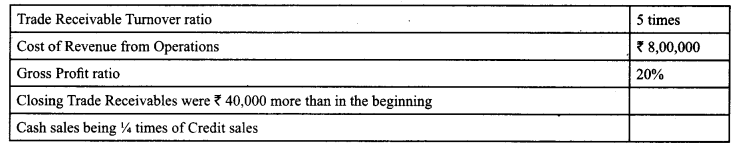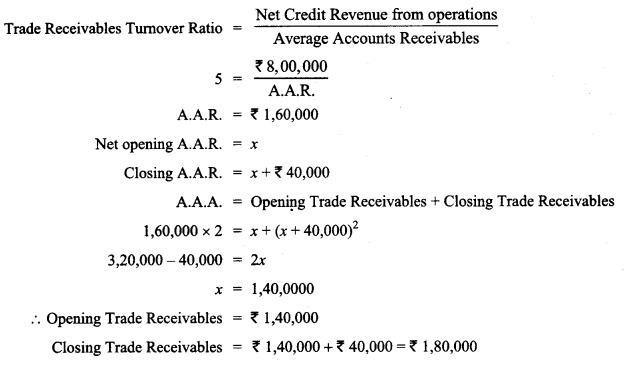Question 17.
(i) What is meant by ‘Activity Ratios’?
(ii) From the following information calculate inventory turnover ratio; Revcnuc from operations 16,00,000; Average Inventory 2,20,000; Gross Loss Ratio 5%. (CBSE Outside Delhi 2016)
(i) Activity Ratio: It refers to the ratio that are calculated for measuring the efficiency of operations of business based on effective utilisation of the resourcess.
Objective of its ratio is to pinpoint the efficiency with which assets are used for generating revenues.
Inventory Turnover Ratio $$\frac { Cost of revenue from operation }{ Average inventory}$$
Cost of revenue from operation = Revenue from operation + Gross loss.
= ₹ 16,00,000 + ₹ 80,000
= ₹ 16,80,000
Average Inventory = ₹ 2,20,000
Inventory Turnover Ratio = $$\frac { ₹ 16,80,000 }{ 2,20,000 }$$
= 7.64 Times.

Question 18.
Calculate Working Capital Turnover Ratio from the following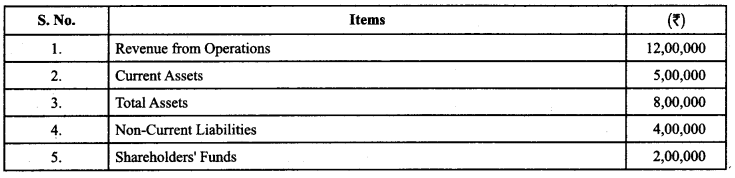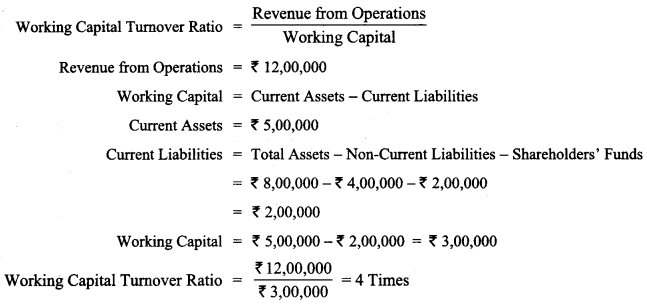Question 19.
Cost of Revenue from Operations = 3,00,000
Inventory Turnover Ratio = 6 Times
Find out the value of Opening Inventory, if opening inventory is 10,000 less than the closing inventory. (CBSE Guidance Notes 2014)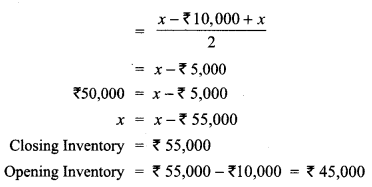Question 20.
(i) What is meant by ‘Profitability Ratios’?
(ii) From the following information calculate inventory turnover ratio; Revenue from operations ₹16,0,000; Average Inventory ₹ 2,20,000; Gross Loss Ratio 5%. (CBSE Outside Delhi 2016)
(i) Profitability Ratio: Profitability ratio are calculated to assess the performance and efficiency of an enterprise. It is to analyse the earning capacity of the firm.

(ii) Inventory Turnover Ratio = $$\frac { Cost of Revenue from operation }{ Average inventory }$$
Cost of Revenue from operation = Revenue from operation + Gross loss.
= 16,00,000 + 80,000 ‘16,80,000
Average Inventory = ‘ 2,20,000
Inventory Turnover Ratio = $$\frac { 18,60,000 }{ 2,20,000 }$$
= 7.64 times.

Question 21.
(i) What is meant by ‘Liquidity of Business’?
(ii) From the following information calculate operating ratio.
Revenue from operations ₹ 6,80,000; Rate of Gross Profit on cost 25%; Selling expenses ₹ 1,44,000; Administrative expenses ₹ 73,000. (CBSE Outside Delhi 2016)
(i) Liqudky of Business: A measure of the extent to which a business has cash to make immediate and short term obligation.
(ii)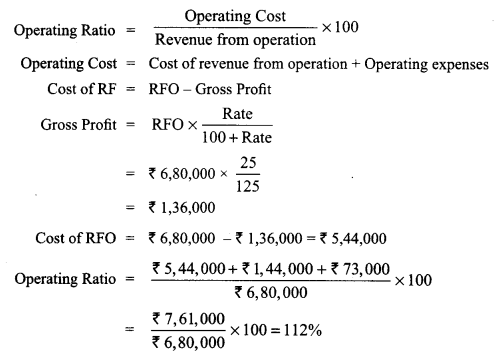Question 22.
(a) X Ltd. has a current ratio of 3.5: 1 and quick ratio of 2: 1. If excess of current assets over quick assets represented by Inventory is 24,000 calculate current assets and current liabilities.

(b) From the following information calculate Inventory Turnover Ratio. Revenue from Operations: 4,00,000 Average Inventory : ‘ 55,000. The rate of Gross Loss on revenue
from Operations was 10%. (CBSE Sample paper Delhi 2016, 2017)
(a)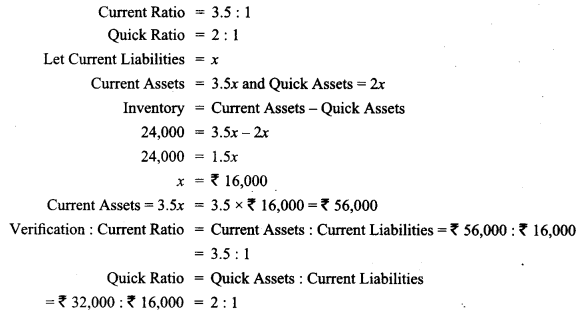(b)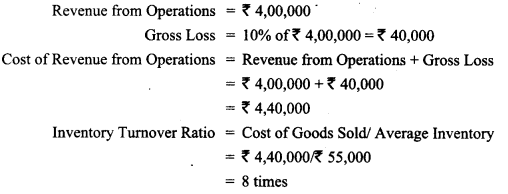Question 23.
From the following caLculate the cross Profit Ratio and Working Capital Turnover Ratio:(a) Gross Profit Ratio = Gross Profit / Net revenue from operations + 100
Gross Profit = Revenue from Operations – Cost of revenue from Operations
= 30,00,000 – 20,00,000 ₹ 10,000
Net Revenue from operations = ₹ 30,00,000
Gross Profit Ratio = 10,00,000 / 30,00,000 x 100 = 33.3%

(b) Working Capital turnover ratio = Net revenue from operations/Working Capital
Net revenue from operations = ₹ 30,00,000
Working Capital = Current Assets – Current Liabilities = 6,00,000 – 2,00,000
= ₹ 4,00,000
Working capital turnover ratio = 30,00,000/4,00,000 = 7.5 times

Question 24.
(a) From the following information, compute ‘Debt-Equity Ratio’:(b) The current ratio of X. Ltd is 2: 1. State with reason which of the following transaction would
(i) increase;
(ii) decrease or
(iii) not change the ratio:
(1) Included in the trade payable was a bills payable of 9,000 which was met on maturity.
(2) Company issued 1,00,000 equity shares of 10 each to the Vendors of machinery purchased. (CBSE Delhi 2014)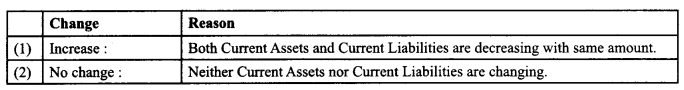Question 25.
From the following calculation:
(a) Net Profit Ratio
(b) Operating Profit Ratio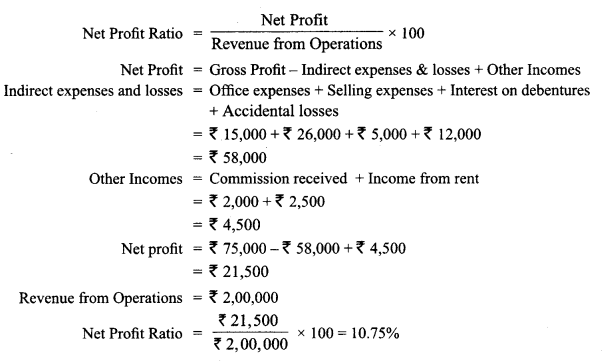(b)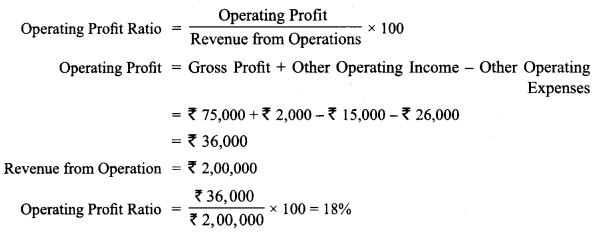Question 26.
The motto of Yash Ltd., an advertising company is ‘Service With Dignity’. Its management and work force is hard-working, honest and motivated. The net profit of the company doubled during the year ended 31.03.2014. Encouraged by its performance company decided to give one month extra salary to all its employees. Following is the Comparative Statement of Profit and Loss of the company for the years ended 31st March 2013 and 2014.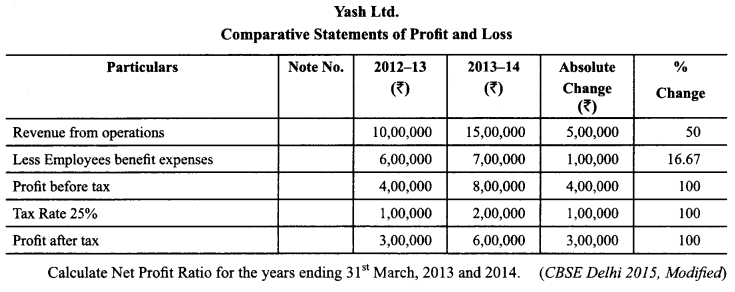Net Profit Ratio = Net Profit after taxi Revenue from operations x 100
As on 31.03.2013 = 3,00,000/10,00,000 x 100
As on 31.03.2014 = 6,00,000/15,00,000 x 100
= 40%

Question 27.
Assume that the Debt-Equity Ratio is 2 : 1. State giving reasons whether this ratio would increase, decrease or remain unchanged in the following cases (Any two):
(a) Purchase of fixed asset on a credit for 2 months.
(b) Purchase of fixed asset on a long-term deferred payment basis.
(c) Issue of new shares for cash.
(d) Issue of bonus shares.
(e) Stile of fixed asset at a loss of ₹3,000. (CBSE 2010 Delhi)
(a) Purchase of fixed asset on a credit for 2 months will not change debt-equity ratio because there is no change in shareholders’ funds.

(b) Purchase of fixed asset on a long-term deferred payment basis will increase debt equity ratio because of increased long-term debts. (Calculated on the basis of liabilities side approach)

(c) Issue of new shares for cash will decrease debt-equity ratio because of increased shareholders’ funds.

(d) Issue of bonus shares will not change debt-equity ratio because of not change because one item of shareholders’ funds has been replaced by another item.

(e) Sale of fixed assets at a loss will increase debt-equity ratio because of reduced shareholders’ funds due to sale of fixed assets at loss.

Question 28.
The gross profit ratio of a company is 50%. State with reason whether the decrease in rent received by ₹ 15,000 will increase, decrease or not change the ratio. (CBSE 2009 Compartment Delhi)
Gross profit ratio will not change because decrease in rent received does not affect gross profit.

Question 29.
The debt equity ratio of Ratan Ltd. is 3 : 1. Giving reasons, state whether the ratio will increase, decrease or not change because of the following transactions:

(i) Issued equity shares of ₹ 1,00,000.
(ii) Discounting a bill of exchange of₹ 50,000 at a discount of 10%.
(iii) Redemption of debentures of₹ 70,000. (CBSE 2013 Compartment OD)
(i) Issue of equity shares will decrease debt-equity ratio because of increased shareholders’ funds.
(ii) Discounting a bill of exchange will increase debt-equity ratio because of lower shareholders’ funds.
(iii) Redemption of debentures will increase debt-equity ratio because proportion of decrease in total debts is lower than proportion of decrease in shareholders’ funds.

Question 30.
The quick ratio of a company is 2 : 1. State giving reasons (for any four), which of the following would improve, reduce or not change the ratio:
(a) Purchase of machinery for cash,
(b) Purchase of goods on credit,
(c) Sale of furniture at cost,
(d) Sale of goods at a profit,
(e) Cash received from debtors. (CBSE 2011 Compartment Delhi)
(a) Purchase of machinery for cash will decrease quick ratio because of lower amount of quick assets.
(b) Purchase of goods on credit will reduce quick ratio because there is no change in quick assets while current liabilities have increased.
(c) Sale of furniture at cost will.improve quick ratio because of increase in quick assets.
(d) Sale of goods at a profit will improve quick ratio because of increase of quick assets.
(e) Cash received from debtors will not change quick ratio because one type of quick asset has been converted into another type of quick asset.

Question 31.
The Quick ratio of a company is 0.8 : 1. State with reason whether the following transactions will increase, decrease or not change the quick ratio :
(1) Purchase of loose tools ₹ 2,000.
(3) Sale of goods on credit t 3,000.
(4) Honoured a bills payable ₹ 5,000 on maturity. (Delhi 2017)

 Transaction Effect on Quick Ratio Reasons (i) Decrease Quick assets have decreased but current liabilities have not changed (ii) Decrease Quick assets have decreased but current liabilities have not changed (iii) Increase Quick assets have decreased but current liabilities have not changed (iv) Decrease Both Quick assets and Current Liabilities have decreased by the same amount

Question 32.
The proprietary ratio of M. Ltd. is 0.80 : 1. State with reasons whether the following transactions will increase, decrease or not change the proprietary ratio :
(i) Obtained a loan from bank ₹ 2,00,000 payable after five years.
(ii) Purchased machinery for cash ₹ 75,000.
(iii) Redeemed 5% redeemable preference shares ₹ 1,00,000.
(iv) Issued equity shares to the vendors of machinery purchased for ₹ 4,00,000. (Outside Delhi 2017)

 Transaction Effect on Quick Ratio Reasons (i) Decrease No change in Shareholders’ funds but total assets will increase by ₹ 2,00,000 (ii) No Change No change in total assets and Shareholders’ funds (iii) Decrease Both Shareholders’ funds and total assets are decreased by same amount (iv) Increase Shareholders’ funds and total assets both are increased

Question 33.
The Current Ratio of a company is 2 : 1. State giving reasons which of the following would improve, reduce or not change the ratio:
(a) Cash paid to trade payables
(b) Sale of fixed tangible assets for cash
(c) Issue of new shares for cash
(d) Payment of final dividend already declared.

 S. No. Effect on Current Ratio Reason {a) Improve Both Current Assets and Current Liabilities have decreased by the same amount (b) Improve Current Liabilities remain unchanged but Current Assets have increased. (c) Improve Current Liabilities remain unchanged but Current Assets have increased. (d) Improve Both Current Assets and Current Liabilities have decreased by the same amount.

Question 34.
The Current Ratio of A Ltd. is 4.5 : 1 and Liquid Ratio is 3: 1. Inventories are 3,00,000. Calculate Current Liabilities. (CBSE Guidance Notes 20M)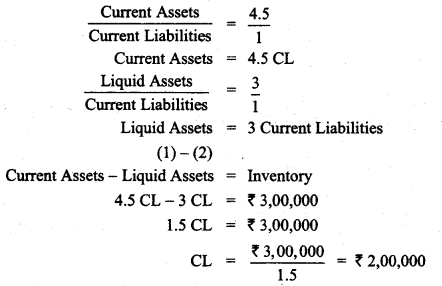Question 35.
From the following information, compute Debt-Equity Ratio: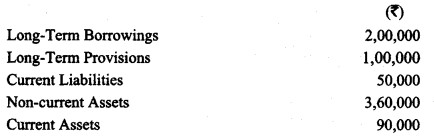Debt-Equity Ratio = $$\frac { Long-term Debts }{ Shareholders Funds }$$
Long-term Debts = Long-term Borrowings + Long-term Provisions
= ₹ 2,00,000 + ₹ 1,00,000 = ₹ 3,00,000
Shareholders’ Funds = Non-current Assets + Current Assets – Long-termBorrowings – Longterm Provisions – Current Liabilities
₹3,60,000 + ₹90,000 – ₹2,00,000 – ₹ 1,00,000 – ₹50,000
= ₹4,50,000 – ₹ 3,50,000 = ₹ 1,00,000

Debt-Equity Ratio = $$\frac { ₹ 3,00,000 }{ ₹ 1,00,000 }$$
= 3:1

Question 36.
From the following information, compute ‘Proprietary Ratio’: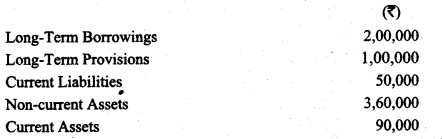Proprietary Ratio = $$\frac { Shareholders’ Funds }{ Total Assets }$$
Shareholders’ Funds = Non-current Assets + Current Assets – Long-term Borrowings – Long-term Provisions – Current Liabilities
= ₹ 3,60,000+₹ 90,000 – ₹ 2,00,000 – ₹ 1,00,000 – ₹ 50,000= ₹ 1,00,000
Total Assets = Non-current Assets + Current Assets
= ₹ 3,60,000 + ₹ 90,000 = ₹ 4,50,000
Proprietary Ratio = $$\frac { ₹ 1,00,000 }{ ₹ 4,50,000 }$$
= 22.22%

Question 37.
Calculate Working Capital Turnover Ratio from the following: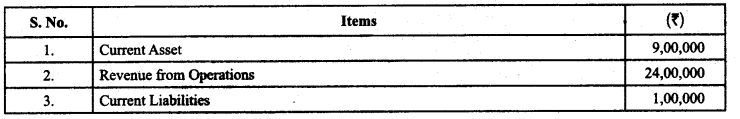Question 38.
For the year ended March 31,2017, Net Profit after tax of K X Limited was ₹ 6,00,000. The company has ₹ 40,00,000 12% Debentures of ₹ 100 each.
(a) Calculate Interest Coverage Ratio assuming 40% tax rate.
(a) Interest Coverage Ratio= Net Profit before Interest and Tax/ Interest on Long-Term Debts
Net Profit after Tax = 6,00,000 Tax Rate = 40%
Net Profit before tax 100/(100 – Tax) x Net Profit after tax
= 100/60 x 6,00,000= 10,00,000
Net Profit before Interest & Tax – Net Profit before tax + Interest on Long-Term Debts
= 10,00,000 + 4,80,000 = 14,80,000
Interest Coverage Ratio = Net Profit before Interest and Tax Interest on Long-Term Debts
= 14,80,000 / 4,80,000 = 3.08 Times

Question 39.
(a) Net profit after interest and tax ₹ 1,00,000; Current assets ₹ 4,00,000; Current liabilities ₹ 2,00,000; Tax rate 20%; Fixed assets ₹ 6,00,000; 10% Long term debt ₹ 4,00,000. Calculate Return on Investment.

(b) Rate of Gross profit on cost of a company is 25%. Its Gross profit is ₹ 5,00,000. Its shareholders’ Funds are ₹ 12,00,000; Current liabilities are ₹ 3,00,000 and Current Assets are ₹ 10,00,000. Calculate its Working Capital Turnover ratio. (Compt. Delhi 2017)Question 40.
With the help of the following information, calculate Return on Investment. Net profit after interest and tax ₹6,00,000; 10% Debentures ₹ 10,00,000; Tax @ 40%; Capital Employed ₹ 80,00,000. (CBSE Compartment Delhi 2015)
Return on Investment = Net Profit before Interest, tax and Dividend/Capital Employed x 100
Net Profit before Tax = 6,00,000 x 100/60 = 10,00,000
Net Profit before Interest, tax and Dividend 10,00,000 + 1 ,00,000 = 11,00,000
Capital Employed = 80,00,000
Return on Investment = 11,00.000/80,00,000 x 100
= 13.45%

Question 41.
Calculate Gross Profit Ratio from the following: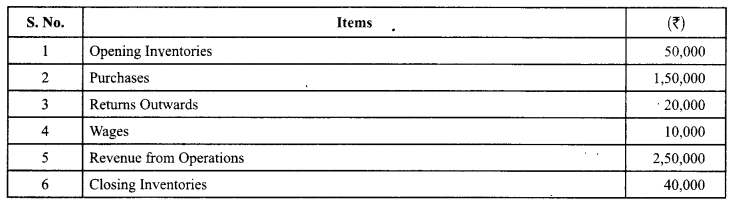Gross Profit Ratio = $$\frac { Gross Profit }{ Revenue from Operations }$$ x 100
Gross Profit = Revenue from Operations – Cost of Revenue from Operations
Cost of Revenue from Operations Opening Inventories + Purchases – Returns outwards + Wages – Closing Inventories
= 50,000 + 1,50,000 – 20,000 + 1,00,000 – 40,000
= 1,50,000
Gross Profit = 2,50,000 – 1,50,000
= 1,00,000

Gross Profit Ratio = $$\frac { 1,00,000 }{ 2,50,000 }$$ x 100 = 40%

Question 42.
From the following Calculate Operating Ratio